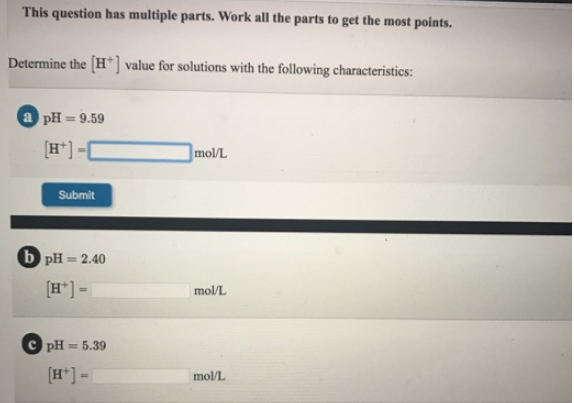Chemistry Practice Problems pH and pOH Practice Problems Solution: The question has multiple parts. Work on all the p...

# Solution: The question has multiple parts. Work on all the parts to get the most points.Determine the [H+] value for solutions with the following characteristics: a. pH = 9.59 b. pH = 2.40 c. pH = 5.39

###### Problem

The question has multiple parts. Work on all the parts to get the most points.

Determine the [H+] value for solutions with the following characteristics:

a. pH = 9.59

b. pH = 2.40

c. pH = 5.39View Complete Written Solution

pH and pOH

pH and pOH

#### Q. What is the pH of a 3.0 M solution of HCIO4?

Solved • Tue Jun 12 2018 12:12:29 GMT-0400 (EDT)

pH and pOH

#### Q. Calculate [H3O+] given [OH -] in each aqueous solution.  Express your answer using two significant figures. Part A[OH -] = 6.1 x 10 -11 M Part BClassi...

Solved • Mon Jun 11 2018 15:44:27 GMT-0400 (EDT)

pH and pOH

#### Q. The pH scale was designed to make it convenient to express hydrogen ion concentrations that are small in aqueous solutions. The definition of pH is in...

Solved • Wed Jun 06 2018 13:12:34 GMT-0400 (EDT)

pH and pOH

#### Q. What are the concentrations of H 3O+ and OH- in oranges that have a pH of 3.76?

Solved • Wed Jun 06 2018 12:37:44 GMT-0400 (EDT)